# Emmy Equating Earthworm Is Here!

Apr 20th 2014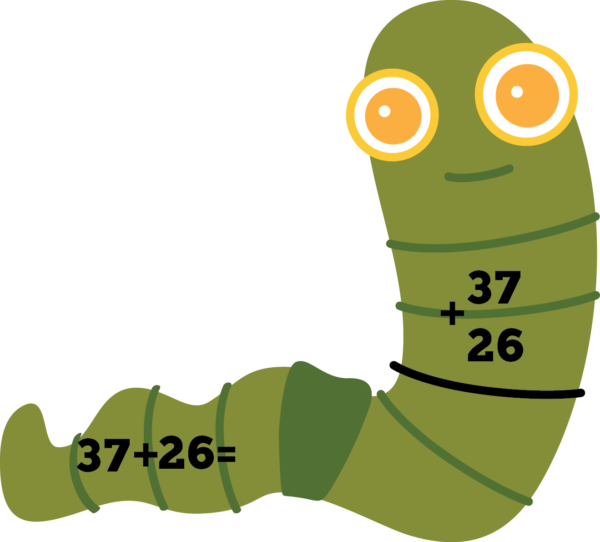Emmy Equating Earthworm here.
Horizontally or vertically, it doesn’t matter the direction,

Emmy Equating Earthworm  is our newest animal in our Problem-Solving Pond: A Common Core Math Strategy Unit. The Problem-Solving Pond was created to help teachers overcome Common Core math challenges and employ problem-solving strategies with confidence and fidelity. Emmy’s unit is perfect for general education, special education, RTI and math intervention. Read more about Emmy’s strategy below or download the complete unit here.

WHAT is an equation? Students write an equation, or number sentence to solve a story problem or show their work after using another method (e.g., drawing a picture, using manipulatives, making a table, etc.).  Solving equations is the very beginning of being able to do algebra. The basic idea behind solving equations is to be able to find the missing number.  Students can use a variety of strategies to solve equations, including traditional algorithms. Traditional algorithms involve repeating a series of steps over and over as in carrying in addition and borrowing in regrouping.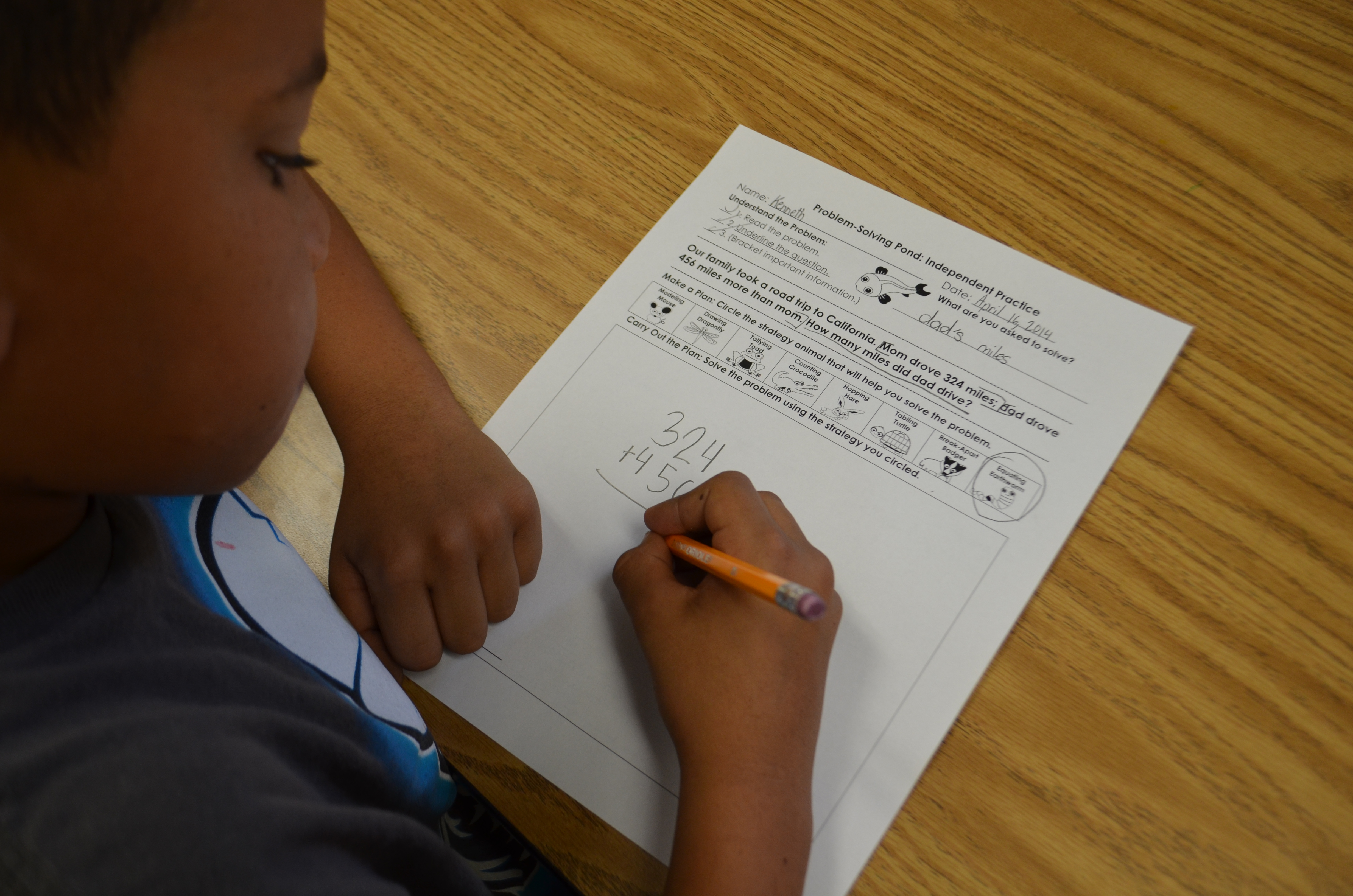WHY are equations important? Traditional algorithms have been the core of many elementary mathematics programs for years as educators focused on quick, precise calculations and paper-pencil drill. Math instruction has drastically changed the last few years with the implementation of Common Core State Standards and the heavy emphasis on science and math instruction. Students are now required to solve real-world math problems using a variety of strategies while explaining thinking and justifying solutions.

Traditional algorithms, or equations, are still acceptable to use as secondary strategies to double-check work and to summarize the mathematics behind the problem-solving process.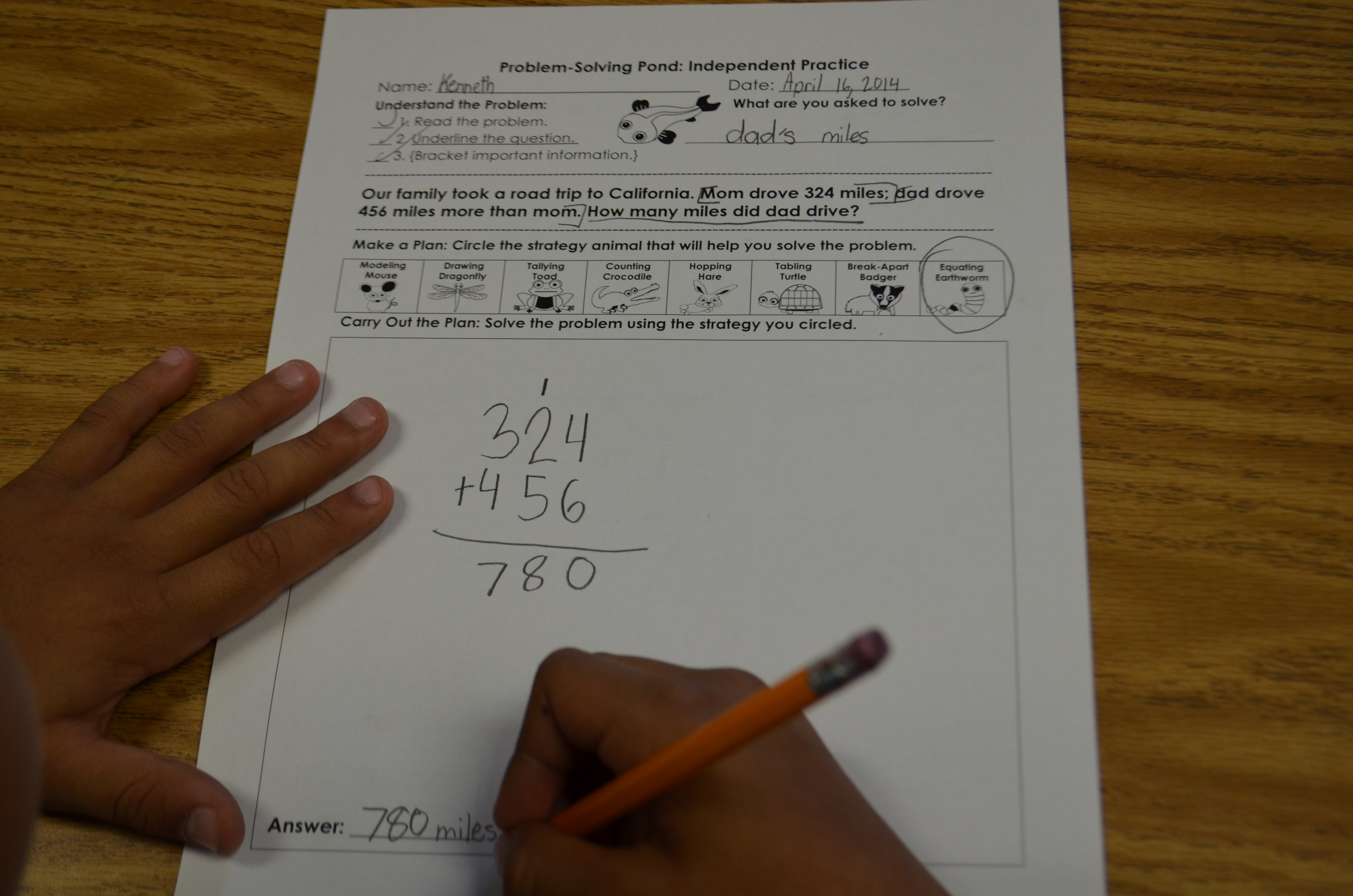HOW do I teach equations? The first major step is to teach students how to find a missing number. This means that students need to be familiar with their basic facts first. Practice a variety of ways to find the missing number using many different equations.  Next, teach addition and subtraction without regrouping; once proficiency is demonstrated, introduce regrouping.

WHEN should I introduce equations? Basic equations and number sentences should be introduced when you begin teaching the problem solving process.  This is also an ideal strategy for proficient mathematicians who have a strong number sense foundation, fluency with basic facts, and are able to quickly conceptualize a problem and use a traditional algorithm to solve.  It is most effective to introduce traditional algorithms after students are able to regroup numbers using place value manipulatives. It is critical that students understand and can articulate the regrouping process before using a traditional algorithm.

Model writing equations and number sentences. Regularly model using think-alouds to demonstrate how equations are used as part of the problem-solving process.  Be explicit in your models to show students how equations can also be used to check their work after using a different strategy to solve the problem.

Provide place value and equation building practice.  Students need a strong place value foundation to use traditional algorithms effectively.  Provide regular practice through center games and kinesthetic activities. Simple games such as “Race to 100” where students roll dice and add numbers using place value manipulatives are engaging and effective.  Incorporate equation building activities into weekly center rotations as well.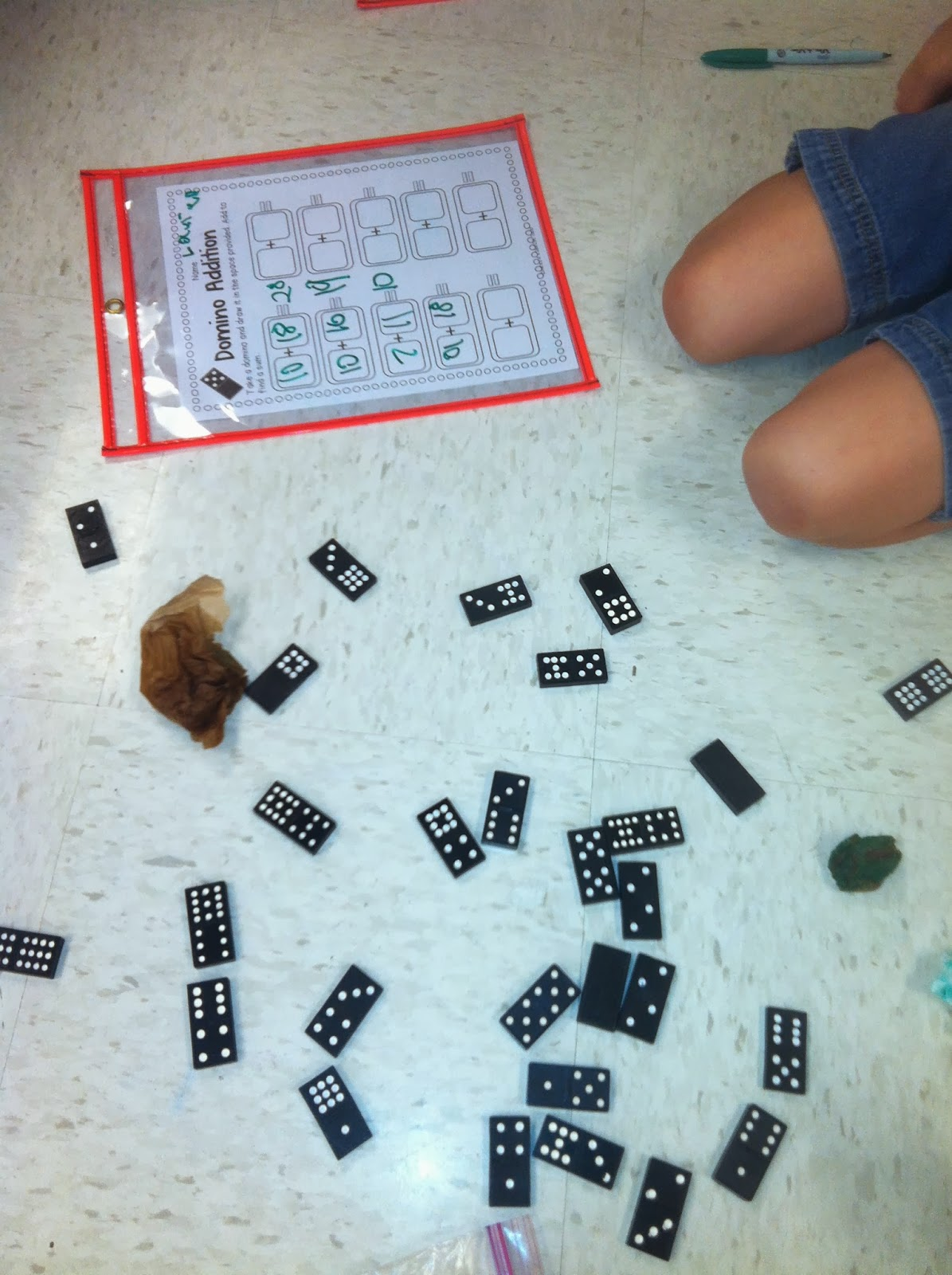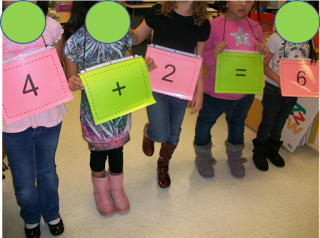Use visual support.  Use the following Subtraction Poem to reinforce regrouping.  Place value and regrouping posters are also helpful tools to post while students are using the traditional algorithm method.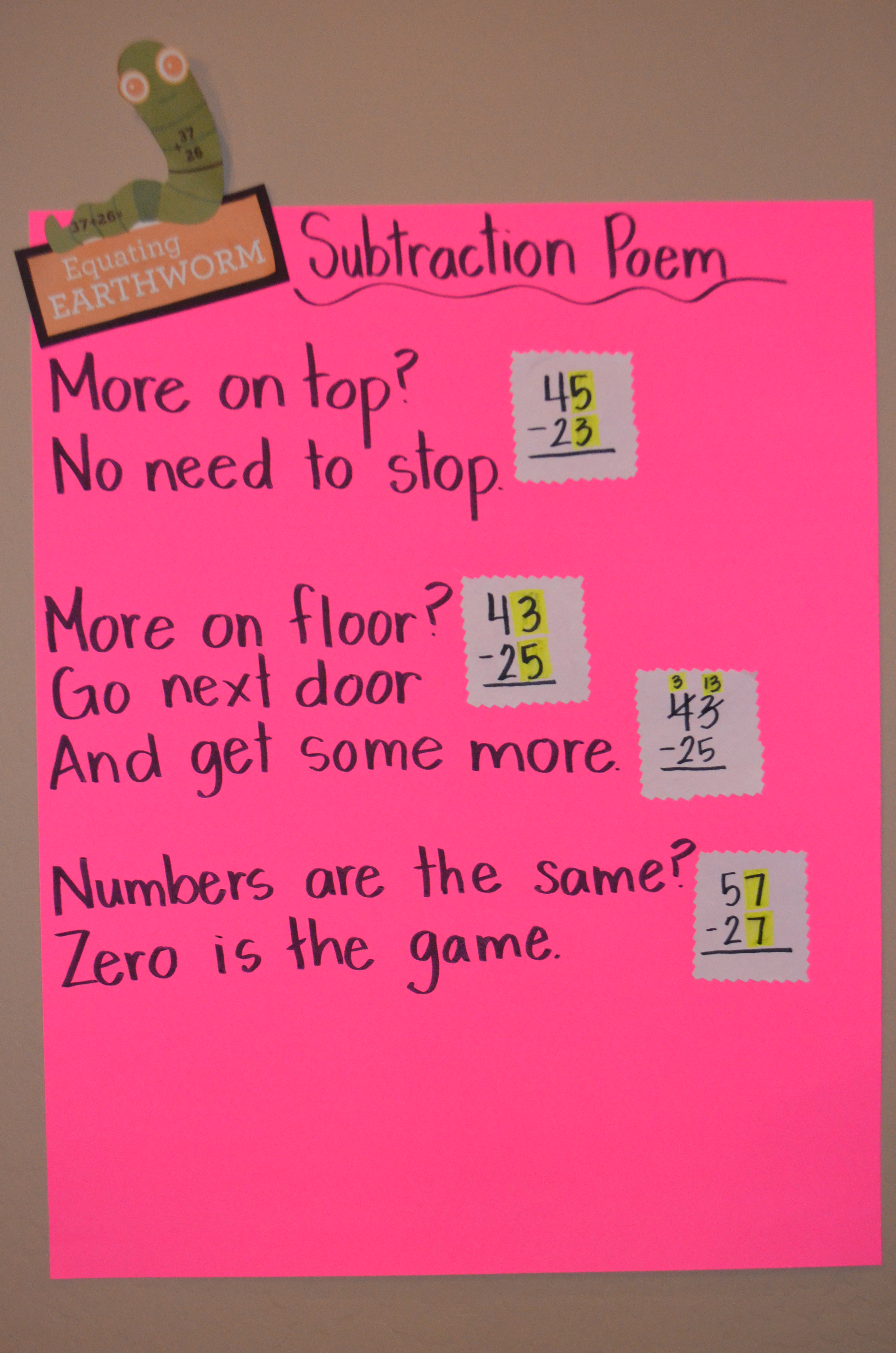Top